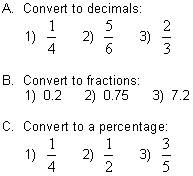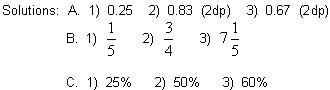# Fractions, Decimals & PercentagesTutorial

math tutorials > fractions, decimals & percentages

A fraction is actually a division.  The numerator is divided by the denominator.

## Fractions and Decimals.

To change a decimal to a fraction you divide the numerator by the denominator.
You may need to use a calculator.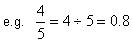To change from a decimal to a fraction the decimal point is removed and the whole number is written over powers of 10.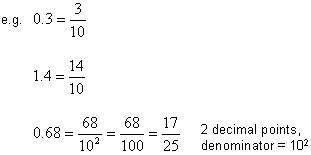## Fractions and Percentages.

Percentages are fractions with a denominator of 100.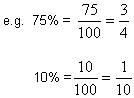To change from a fraction to a percentage, multiply the fraction by 100%.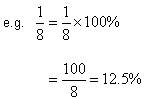## Fraction and decimal problems for you to try.